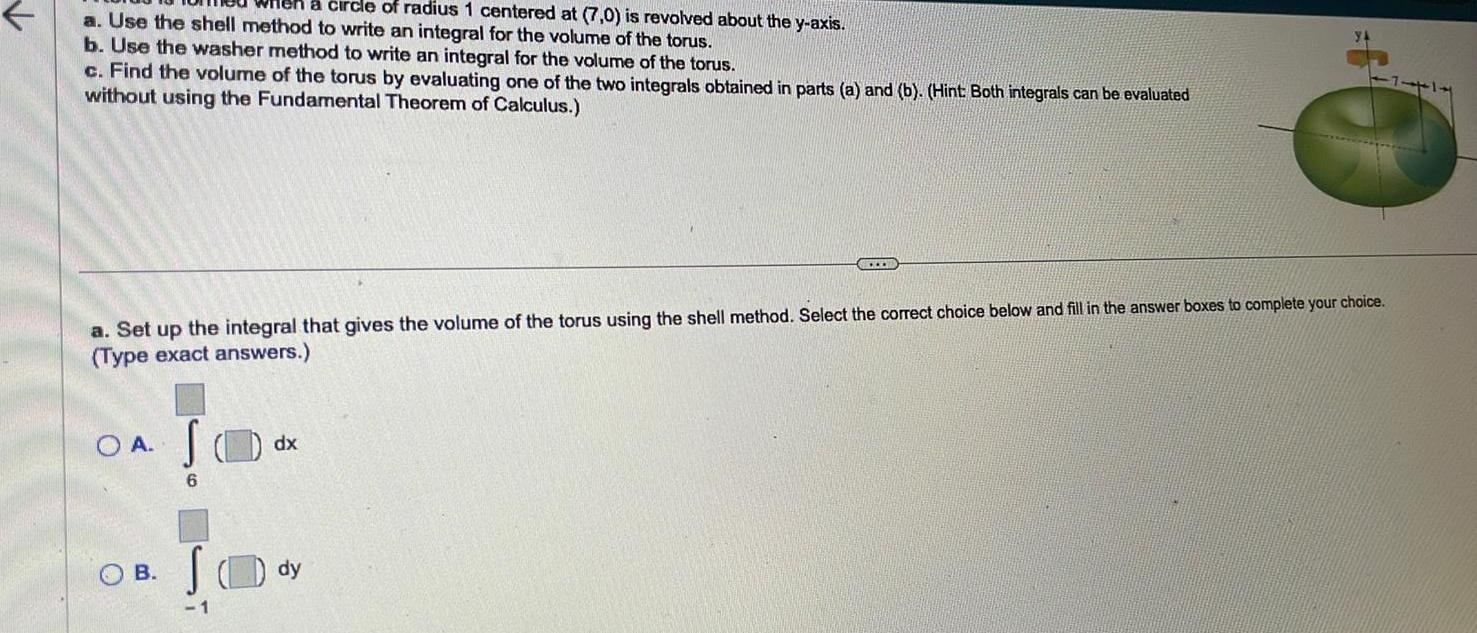Question:

# a circle of radius 1 centered at 7 0 is revolved about the y

Last updated: 11/19/2023a circle of radius 1 centered at 7 0 is revolved about the y axis a Use the shell method to write an integral for the volume of the torus b Use the washer method to write an integral for the volume of the torus c Find the volume of the torus by evaluating one of the two integrals obtained in parts a and b Hint Both integrals can be evaluated without using the Fundamental Theorem of Calculus a Set up the integral that gives the volume of the torus using the shell method Select the correct choice below and fill in the answer boxes to complete your choice Type exact answers O A B S 6 1 dx dy